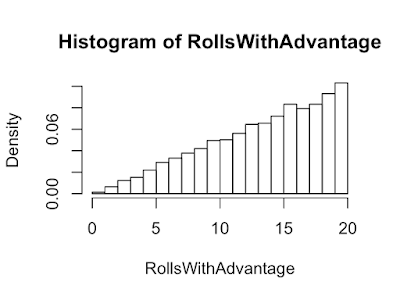## Wednesday, June 24, 2020

### Dungeons and Dragons: Advantage

#### D20 and Random Events

In the game Dungeons and Dragons, the success or failure of an event is determined by rolling a 20 sided die (D20): higher is better. If you need to roll 11 or higher you have a 50% chance of success. If another event requires 10 or better you now have a 55% chance for success. Each point the roll goes up or down is worth 5%. Often times, rolling a 20 is a critical success and a 1 is a critical failure; critical means it's extra good/bad.

Sometimes things are really going your way and you roll with advantage. Sometimes things are not in your favor and you roll with disadvantage. When rolling with advantage, roll two dice and pick the higher. When rolling with disadvantage, roll two dice and pick the lower. How do advantage and disadvantage affect the chance of getting a 20 or 1?

When rolling without advantage or disadvantage, the probability of getting a 20 or a 1 is 1/20 = 0.05 or 5%. The probability of not getting a twenty 1 - 1/20 = 19/20.

```P20    <- 0.05
Pnot20 <- 1-P20
```

When you have advantage, to not get a 20, you have to not roll a 20 twice. The probability of getting a 20 is (1 - the proability of not getting a 20 twice), and as you can see below, is almost 10%. Advantage about doubles you chance of getting a 20. But you probability guessed that since you're rolling twice. :) The chance of getting a 1 is 0.05^2=0.0025, almost 0!

```Pnot20*Pnot20
```
```##  0.9025
```
```1 - Pnot20*Pnot20
```
```##  0.0975
```

How will advantage and disadvantage affect the average roll? This time let's estimate the answer using a simulation. A 1000 trials will give a good estimate.

```Trials <-10000
```

Below we simulate rolling two D20s

```set.seed(1)
x <- sample(1:20,Trials,replace = TRUE)
y <- sample(1:20,Trials,replace = TRUE)
```

When we have advantage, roll two D20 and pick the max. With disadvantage roll two D20 and pick the min.

```RollsWithAdvantage <- apply(cbind(x,y), 1,max)
RollsWithDisadvantage <- apply(cbind(x,y), 1,min)
```

Below is the simulated advantage mean, the calculated mean of a regular D20 roll, and the simulated disadvantage mean. Advantage adds about 3.4 points and disadvantage subtracts 3.4 or about +/- 17%.

```Advantage
```
```##  13.8863
```
```mean(1:20)
```
```##  10.5
```
```Disadvantage
```
```##  7.1287
```

Below are 3 figures with histograms. The first histogram is made with 10,000 simulated D20 advantage rolls. Advantage moves a lot of probability to the right. The second histogram is made using 10,000 simulated D20 regular rolls. The histogram is approximately flat and each number is at about 0.05% and that's what we calculated. The last histogram is made with 10,000 simulated D20 disadvantage rolls. Disadvantage moves an equal amount to the left.The probability of getting 11 or better with advantage is 1- probability of getting 10 or less twice. Rolling with advantage moves a 50% chance to a 75% chance!

```p10orLess <- 0.5
1 - p10orLess^2
```
```##  0.75
```

#### 1 comment:

1.Which you have shared here. Your article is very informative and nicely describes the process . Thank you so much. dnd würfel set